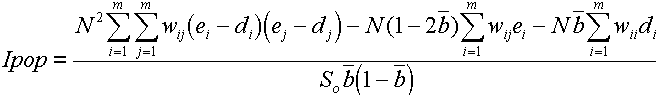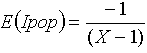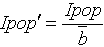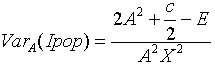﻿ Ipop: Statistic

# Oden's Ipop: Statistic

 Ho Disease rates in connected areas are independent. The geographic variation in the number of cases is expected to follow geographic variation in population size. Ha Disease rates in connected areas are not spatially independent. Geographic variation in the number of cases does not follow geographic variation in population size

## Test Statistic

Moran's I (Moran 1950) is a weighted correlation coefficient used to detect departures from spatial randomness. Moran's I is used to determine whether neighboring areas are more similar than would be expected under the null hypothesis. Oden (1995) adjusted Moran's I to account for differences in population size across areas. Use Ipop when population size data are available.

Ipop requires a fair amount of notation. In essence, it is large when there is clustering within a region or among adjacent regions.m represents the number of locations or areas N is the total number of cases in all of the areas ni is the total number of cases in area i ei is the proportion of cases in area i (ei = ni/N). X is the total size of the risk population in all areas xi is the size of the risk population in area i di is the proportion of the population in area i, di = xi/X ei - di is the difference between the proportion of cases in area i and the number of cases expected given the area's population size.  b is the average prevalence, b = N/X, b2 = 1/b(1-b) -3. S0 = X2A-XB, S1 = X3E-4X2F+4XD wij is a weight denoting the strength of connection between areas i and j, developed from neighbor information.The expectation of Ipop under the null hypothesis (no clustering) approaches zero for large total population:The range of Ipop depends on population size, therefore t can be useful to standardize the statistic using the average prevalence, for comparison among different study areas.The variance of Ipop can be determined based on a random distribution, appropriate for disease rates (Cliff and Ord 1981). ClusterSeer calculates the variance in two ways. The variance of Ipop under the null hypothesis is:It also calculates an approximation of the variance (VarA):## Significance

ClusterSeer evaluates the significance of Ipop using three approaches: using the z-scores and variance calculated in each way and through Monte Carlo randomization, using multinomial randomization. In general, these three methods will report relatively similar p-values. The approximation and randomization assumption methods are only valid when the data can be assumed to be distributed normally. When the data may not be normally distributed, use the Monte Carlo p-value instead.